# 3D Polygon - draw planes with Matlab

In this example we’re going to explain how to draw a 3D polygon using Matlab. More specifically, we are going to draw several flat surfaces (planes) to build or model a box.

Our main built-in function in this case is the function “fill3”, which is intended to color 3D flat planes.

The sentence fill3(x, y, z, c) would fill the 3D polygon defined by vectors x, y and z with the color specified by c.  The vertices of the polygon are specified by coordinates of components of x, y and z, and the polygon is closed by connecting the last vertex to the first one.

Let’s say that we want to draw this arbitrary rectangular boxThe coordinates of the eight vertices are as follows:

P1(0, 0, 0)
P2(2, 0, 0)
P3(2, 4, 0)
P4(0, 4, 0)
P5(0, 0, 3)
P6(2, 0, 3)
P7(2, 4, 3)
P8(0, 4, 3)

We could draw our first flat surface by filling the area defined by the first four points, like this:

p1 = [0 0 0];
p2 = [2 0 0];
p3 = [2 4 0];
p4 = [0 4 0];

x = [p1(1) p2(1) p3(1) p4(1)];
y = [p1(2) p2(2) p3(2) p4(2)];
z = [p1(3) p2(3) p3(3) p4(3)];

fill3(x, y, z, 1);
xlabel(
'x'); ylabel('y'); zlabel('z');

The code produces this figure: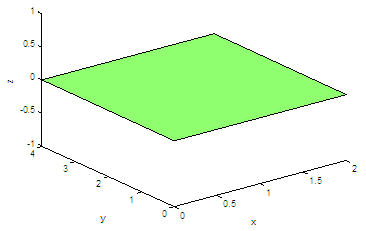We can expand our axes, add a grid and hold the image in order to add more planes...

axis([-1 3 -1 5 -1 4])
grid
hold
on

produces...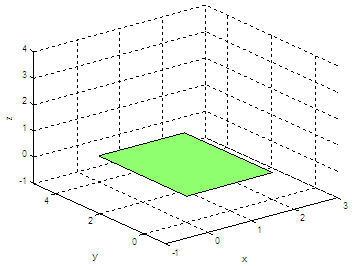We can draw a second surface or plane defined by the last four vertices:

p5 = [0 0 3];
p6 = [2 0 3];
p7 = [2 4 3];
p8 = [0 4 3];

x = [p5(1) p6(1) p7(1) p8(1)];
y = [p5(2) p6(2) p7(2) p8(2)];
z = [p5(3) p6(3) p7(3) p8(3)];
fill3(x, y, z, 2);

And the result now is: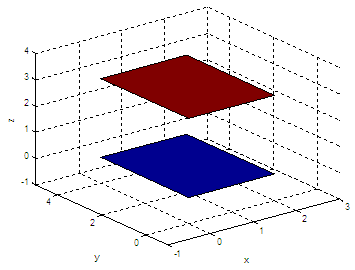We can add more surfaces by connecting the appropriate vertices, in the correct order. If we continue our code above with

x = [p2(1) p6(1) p7(1) p3(1)];
y = [p2(2) p6(2) p7(2) p3(2)];
z = [p2(3) p6(3) p7(3) p3(3)];
fill3(x, y, z, 3);

x = [p2(1) p6(1) p5(1) p1(1)];
y = [p2(2) p6(2) p5(2) p1(2)];
z = [p2(3) p6(3) p5(3) p1(3)];
fill3(x, y, z, 4);

The resulting 3D-image is the following figure: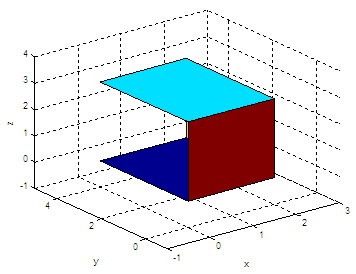We can produce or generate different views of the same 3D-image, of course:

view(120, 50)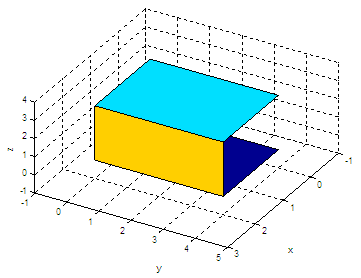Naturally, we can have a custom-made function to draw rectangles. For example, save something like this:

function poly_rectangle(p1, p2, p3, p4)
% The points must be in the correct sequence.
% The coordinates must consider x, y and z-axes.

x = [p1(1) p2(1) p3(1) p4(1)];
y = [p1(2) p2(2) p3(2) p4(2)];
z = [p1(3) p2(3) p3(3) p4(3)];
fill3(x, y, z, rand(size(p1)));
hold on

We can call that function from any other script or from the command window.

Let’s try it:

poly_rectangle(p3, p4, p8, p7)
poly_rectangle(p1, p4, p8, p5)
view(-155, 43)

What did you get?

From '3D Polygon' to Matlab home

From '3D Polygon' to 3D figures